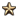####We are conducting a survey to learn how we can improve the Paradox Wikis experience for our users. Please take the time to fill the SurveyTrade could be implemented with cycles fairly easily (as long as merchant boost was removed to avoid potentially infinite trade value).

## Absorbing Markov chain

Based on posts by forum user Phi , this can be represented as a absorbing Markov chain.

First, arrange the matrix Q = I - L, where L is the weighted Laplacian matrix of the following graph:

• Take the trade node graph. These vertices Vt represent trade value in the trade network. Weight the edges according to the trade value share steered on that edge.
• For every trade node, add an adjacent vertex Vc representing trade value that has been collected at that node. Weight the edge according to the collecting trade value share at that trade node.

For example, say we have three trade nodes A, B, and C, where:

• At A, 20% is sent to B, 30% is sent to C, and 50% is collected.
• At B, 10% is sent to A, 30% is sent to C, and 60% is collected.
• At C, 20% is sent to A, 40% is sent to B, and 40% is collected.

The matrix looks like this:

At Bt Ct Ac Bc Cc
At 0.1 0.2
Bt 0.2 0.4
Ct 0.3 0.3
Ac 0.5 1.0
Bc 0.6 1.0
Cc 0.4 1.0

The first three columns are just translations of what I wrote above. The last three columns represent that collected trade stays collected. We can then solve this as for an absorbing Markov chain . To ensure absorption, we could add a token drain at each node (call it "pirates" or some such).

### Incoming and outgoing

To figure out the incoming and outgoing trade, observe that local + incoming = outgoing + collected. We know the local and collected trade value at this point. Furthermore, we know the ratio of outgoing and collected from the trade value shares. So we have two equations and two unknowns, which we can solve for incoming and outgoing. Namely:

```incoming = collected / collecting trade power share - local
outgoing = local + incoming - collected
```

For this example, given local values of 20, 30, and 10 for A, B, and C respectively, we get:

Node Incoming Outgoing
A 11.9588 15.9794
B 20.2577 20.1031
C 24.6649 20.7990

The user can then verify that these values reflect the trade value shares.

## Code

MATLAB:

```function [ collected, incoming, outgoing ] = tradeWithCycles( network, collectionShares, localValues )
% network: n x n matrix representing the trade network
% localValues: n x 1 vector representing local trade value at each node
% collectionShares: n x 1 vector representing proportion collected at each node
B = diag(collectionShares) / (eye(size(network)) - network);
collected = B * localValues;
incoming = collected ./ collectionShares - localValues;
outgoing = localValues + incoming - collected;
end
```

## Performance

On my machine (Intel Core i5 760 @ 2.8 GHz) the following test code

```n=58;
numTrials=10000;
tic;
for i = 1:numTrials
[collected, incoming, outgoing] = gaming.eu4.tradeWithCycles(rand(n), rand(n, 1), rand(n, 1));
end
elapsed = toc;
elapsed / numTrials
```

results in a time of less than 0.3 ms per trial. In other words, at this rate performing this computation once per month for the entire game span would take less than 2 seconds. Of course, there may be implementation complications in practice; for example Paradox seems to represent numbers as thousandths stored in a 32-bit integer rather than using doubles like MATLAB.